# IB DP Chemistry: SL复习笔记8.2.7 Strong & Weak Acids & Bases

### Strong & Weak Acids & Bases

#### Strong acids

• strong acid is an acid that dissociates almost completely in aqueous solutions
• HCl (hydrochloric acid), HNO3 (nitric acid) and H2SO4 (sulfuric acid)
• The position of the equilibrium is so far over to the right that you can represent the reaction as an irreversible reaction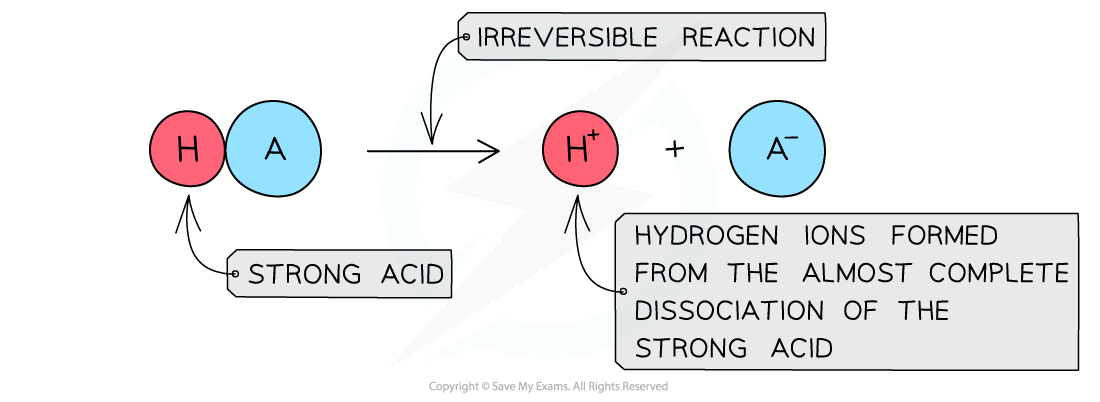The diagram shows the complete dissociation of a strong acid in aqueous solution

• The solution formed is highly acidic due to the high concentration of the H+/H3O+ ions
• Since the pH depends on the concentration of H+/H3O+ ions, the pH can be calculated if the concentration of the strong acid is knownpH is the negative log of the concentration of H+/H3O+ ions and can be calculated if the concentration of the strong acid is known using the stoichiometry of the reaction

#### Weak acids

• weak acid is an acid that partially (or incompletely) dissociates in aqueous solutions
• Eg. most organic acids (ethanoic acid), HCN (hydrocyanic acid), H2S (hydrogen sulfide) and H2CO3 (carbonic acid)
• The position of the equilibrium is more over to the left and an equilibrium is established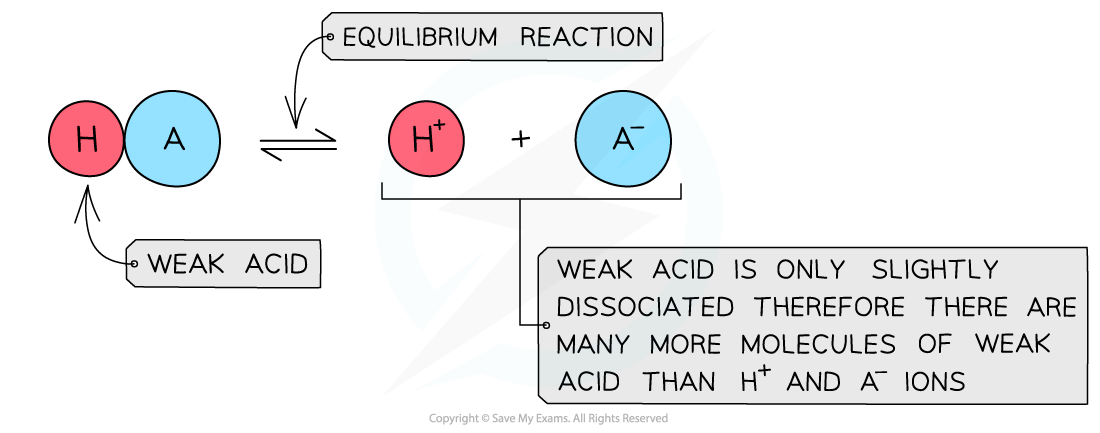The diagram shows the partial dissociation of a weak acid in aqueous solution

• The solution is less acidic due to the lower concentration of H+/H3O+ ions
• Finding the pH of a weak acid requires using the acid dissociation constant, Kbut this not required at Standard Level, but only at Higher Level and is covered in Topic 18

Acid & Equilibrium Position Table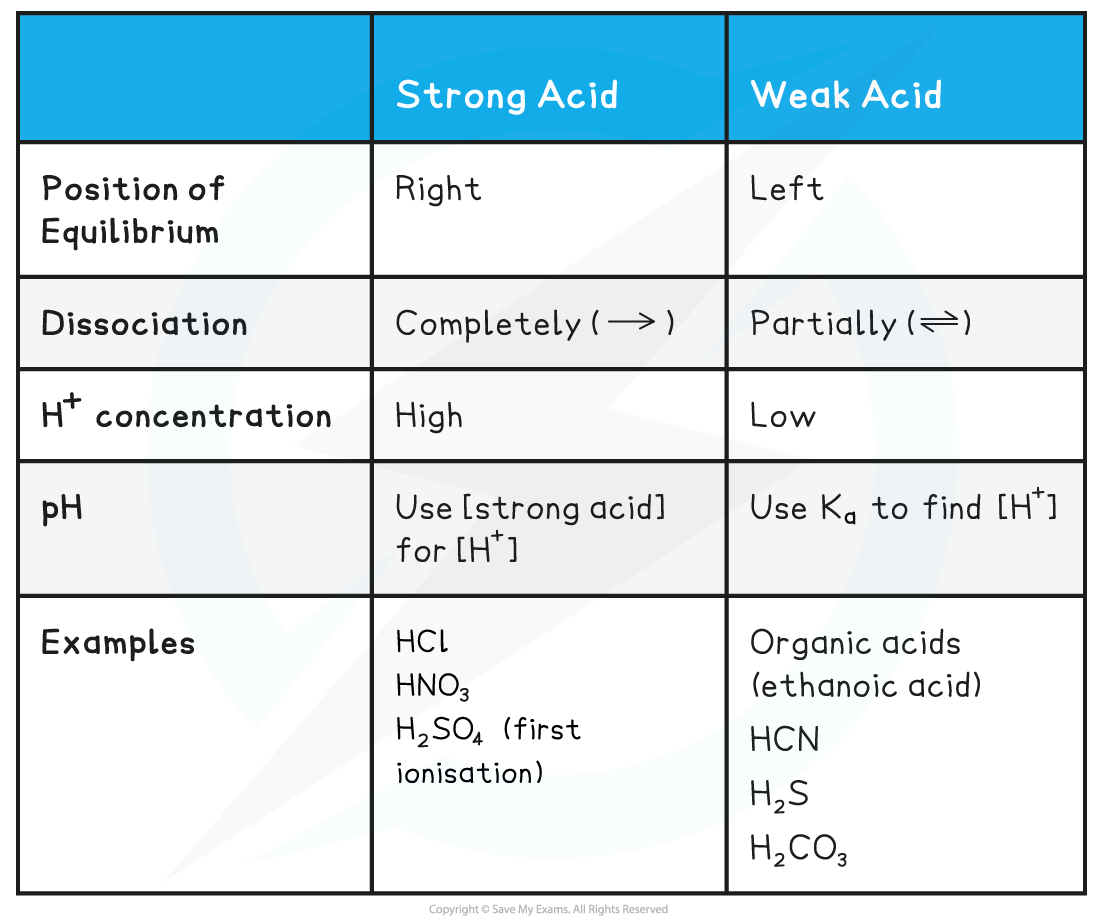#### Strong bases

• strong base is a base that dissociates almost completely in aqueous solutionsE.g. group 1 metal hydroxides such as NaOH (sodium hydroxide)
• The position of the equilibrium is so far over to the right that you can represent the reaction as an irreversible reaction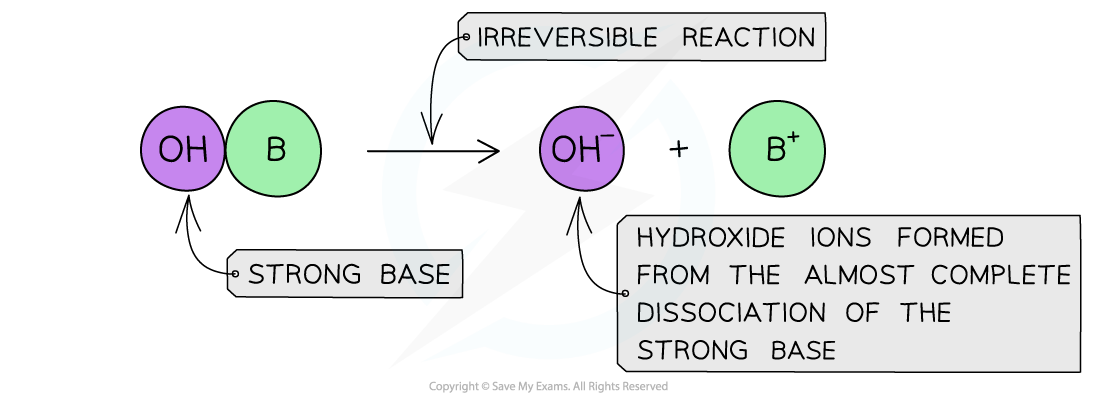The diagram shows the complete dissociation of a strong base in aqueous solution

• The solution formed is highly basic due to the high concentration of the OH- ions

#### Weak bases

• weak base is a base that partially (or incompletely) dissociates in aqueous solutions
• NH3 (ammonia), amines and some hydroxides of transition metals
• The position of the equilibrium is more to the left and an equilibrium is established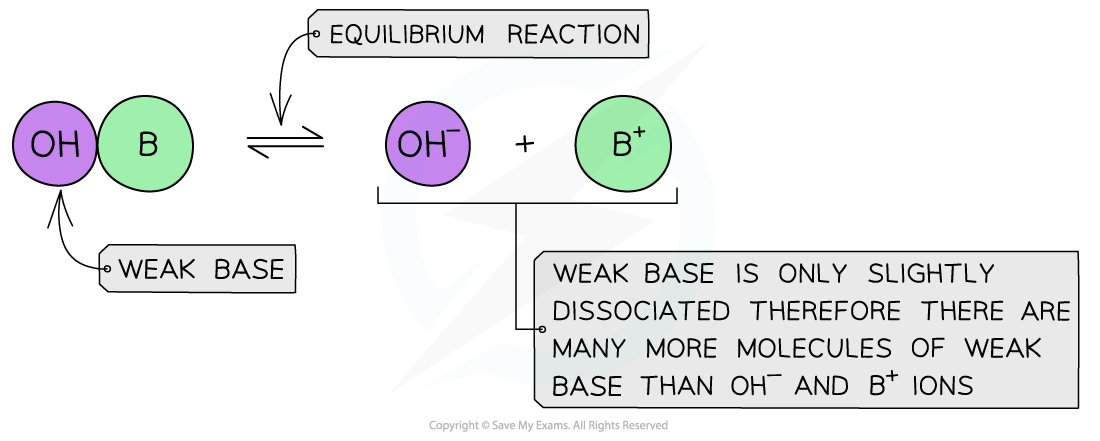The diagram shows the partial dissociation of a weak base in aqueous solution

• The solution is less basic due to the lower concentration of OH- ions

Base & Equilibrium Position Table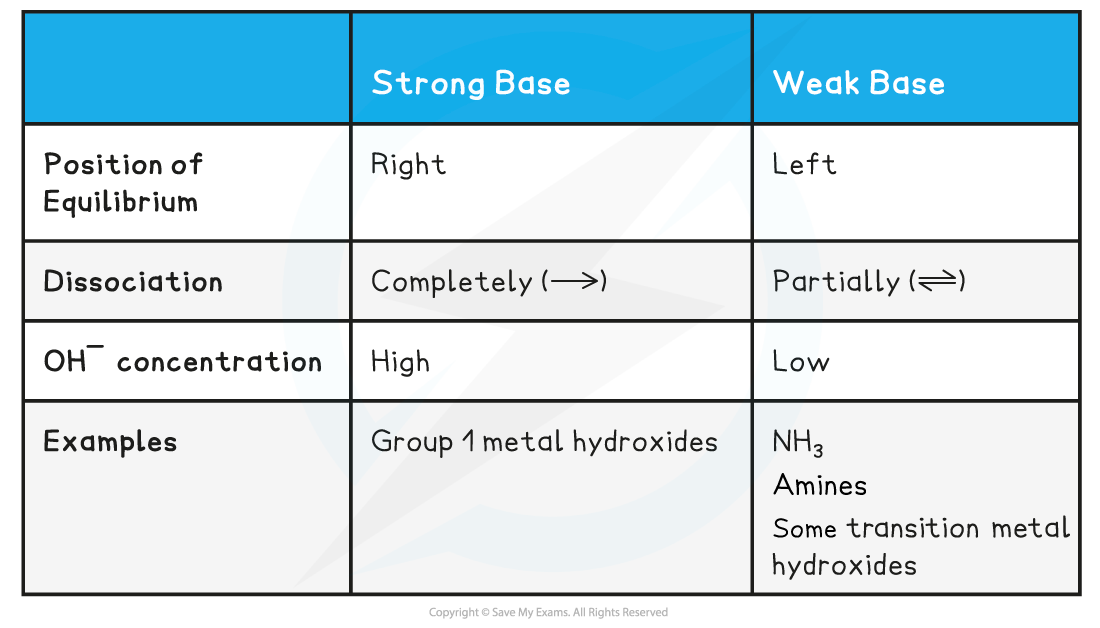### Conjugate Pairs & Acid-Base Strength

• The conjugate base of HCl is the chloride ion, Cl-, but since the reverse reaction is virtually non-existent the chloride ion must be a very weak conjugate base

HCl (g) → H(aq)  +   Cl(aq)

acid                          conjugate base

• In general strong acids produce weak conjugate bases and weak acids produce strong conjugate bases
• A strong base is also fully ionized and is a good proton acceptor
• For example the hydroxide ion is a strong base and readily accepts protons:

OH(aq)+  H+ (aq)  ⇌  H2O (l)

• The conjugate acid of the hydroxide ion is water, which is a weak conjugate acid
• In general strong bases produce weak conjugate acids

#### Exam Tip

Hydrogen ions in aqueous solutions can be written as either as H3O+ or as Hhowever, if H3O+ is used, H2O should be included in the chemical equation: HCl(g) → H+(aq) + Cl-(aq)   OR HCl(g) + H2O(l) → H3O+(aq) + Cl-(aq) Some acids contain two replaceable protons ( called 'dibasic') – for example, H2SO4 (sulfuric acid) has two ionisations: H2SO4 acts as a strong acid: H2SO4 → H+ + SO4-HSO4- acts as a weak acid: HSO4- ⇌ H+ SO42-The second ionisation is only partial which is why the concentration of 1 mol dm-3 sulfuric acid is not 2 mol dm-3 in H+ ions Also, don't forget that the terms strong and weak acids and bases are related to the degree of dissociation and not the concentration.The appropriate terms to use when describing concentration are dilute and concentrated.